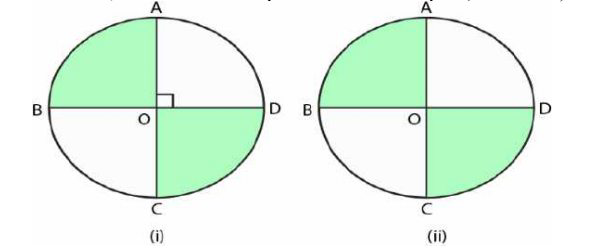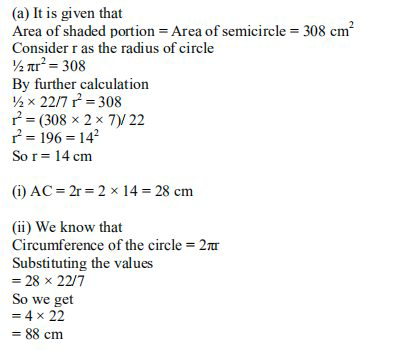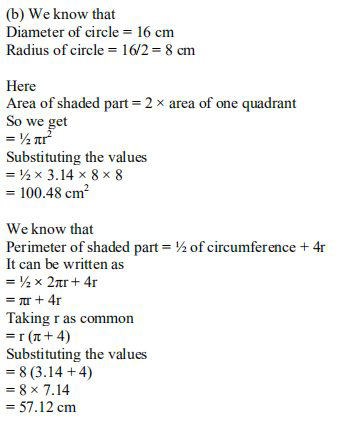# ML Aggarwal Solutions Class 9 Mathematics Solutions for Mensuration Exercise 16.3 in Chapter 16 - Mensuration

(a) In the figure (i) given below, AC and BD are two perpendicular diameters of a circle ABCD. Given

that the area of the shaded portion is 308 \mathrm{cm}^{2}

calculate

(i) the length of AC and

(ii) the circumference of the circle

(b) In the figure (ii) given below, AC and BD are two perpendicular diameters of a circle with center O. If

AC = 16 cm, calculate the area and perimeter of the shaded part. (Take π = 3.14)The route or boundary that surrounds any shape is defined by its circumference. In other terms, the circumference is also known as the perimeter, and it aids in determining the length of any shape's contour.Related Questions

Lido

Courses

Teachers

Book a Demo with us

Syllabus

Maths
CBSE
Maths
ICSE
Science
CBSE

Science
ICSE
English
CBSE
English
ICSE
Coding

Terms & Policies

Selina Question Bank

Maths
Physics
Biology

Allied Question Bank

Chemistry
Connect with us on social media!